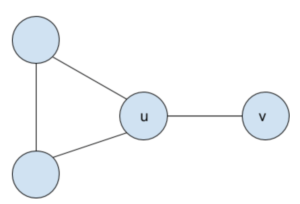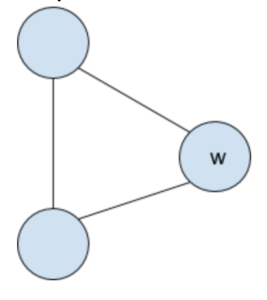# Introduction

This equation is what we are trying to solve here.

G is the Graph and is the number of color available.

If you remember how to calculate derivation for function, this is the same principle here. You need to look at your Graph and isolate component and use formula that you need to remember by heart. We will explain them later in this article.

## Definition

Vertices is a point or a node of the graph.

Edge is a connection between two Vertices.

# List of the Chromatic Polynomial formulas with simple graphs

## When graph have 0 edge

Where n is the number of Vertices.

Python Code:

def chromatic_polynomial(lambda, vertices):
return lambda ** vertices

## Complete Graph

This is a graph which have each of its Vertices connected to all the other Vertices.

Which mean that

Where E is the number of Edges and V the number of Vertices.

The Chromatic Polynomial formula is:

Where n is the number of Vertices.

Python Code:

import math
def chromatic_polynomial(lambda, vertices):
return math.factorial(lambda) / math.factorial(lambda - vertices)

## For Path

A path is graph which is a “line”. Each Vertices is connected to the Vertices before and after it. This graph don’t have loops, and each Vertices is connected to the next one in the chain.

So

Where E is the number of Edges and V the number of Vertices.

The Chromatic Polynomial formula is:

Where n is the number of Vertices.

Python Code:

def chromatic_polynomial(lambda, vertices):
return lambda * ( ( lambda - 1) ** (vertices - 1) )

## For Cycle / Loop

A cycle or a loop is when the graph is a path which close on itself.

That mean that:

Where E is the number of Edges and V the number of Vertices.

The Chromatic Polynomial formula is:

Where n is the number of Vertices.

Python Code:

def chromatic_polynomial(lambda, vertices):
return ( lambda - 1 ) ** vertices + (( -1 ) ** vertices) * ( lambda - 1 )

## Composed Graph

This is when you have two graph which are not connected by edges.

The Chromatic Polynomial formula is:

Where is the Graph number i . And M is the number of Graphs.

Python Code:

from functools import reduce
def chromatic_polynomial(lambda, vertices, graph_chromatic_polynomial*):
return reduce(operator.mul, map(lambda f: f(lambda, vertices), graph_chromatic_polynomial), 1)

# Combining formulas

Here is when it gets interesting. This is when you combine the formulas together to get the final polynomial formula.

The same way that derivative have rules to combine formulas, there are some here as well.

For two vertices u and v .

is the Graph G with the Edge between u and v removed.

is the Graph G with the Edge between u and v added.

is the Graph G with the vertices u and v combined into one vertex.

Then here is how you can modify graph to transform them into any shape from above:

So you can recursively add, remove and combine vertices to reach shapes that you know.

## Example

With a given graph G:You will get:

### G – uvHere you can see one loop, with the formula:

with n is 3 here:

The unconnected vertices formula is:

Where n here is 1:

Composed:

### G/uv

After combining u and v, we get:Which is just a loop:

With 3 vertices:

# To know more

https://www.wikiwand.com/en/Chromatic_polynomial

http://mathworld.wolfram.com/ChromaticPolynomial.html